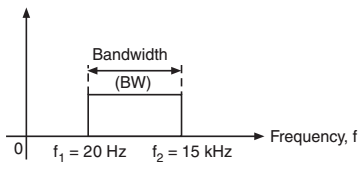# What is Bandwidth?

## Bandwidth

We can define Bandwidth as the portion of the electromagnetic spectrum occupied by a signal.

It can also be defined as the frequency range over which an information signal is transmitted.

Bandwidth can be calculated as the difference between the upper and lower frequency limits of the signal.

As we already know there are different types of passband signals such as voice signal, music signal, TV signal, etc. Each of these signals have its own frequency range. This frequency range of a signal is known as its bandwidth.

For example, the range of music signal is 20 Hz to 15 kHz.Figure.1 : Bandwidth of music signal

Therefore, as shown in figure 1, the bandwidth is = f2 – f1

Thus,  BW =  f2 – f1

BW = 15000 – 20 = 14980 Hz

The bandwidth of different signals are listed in table 1 below.Note : The required bandwidth in the data transmission actually depends upon the rate at which the data is being transmitted. The BW increases with increase in the rate of data transmission.